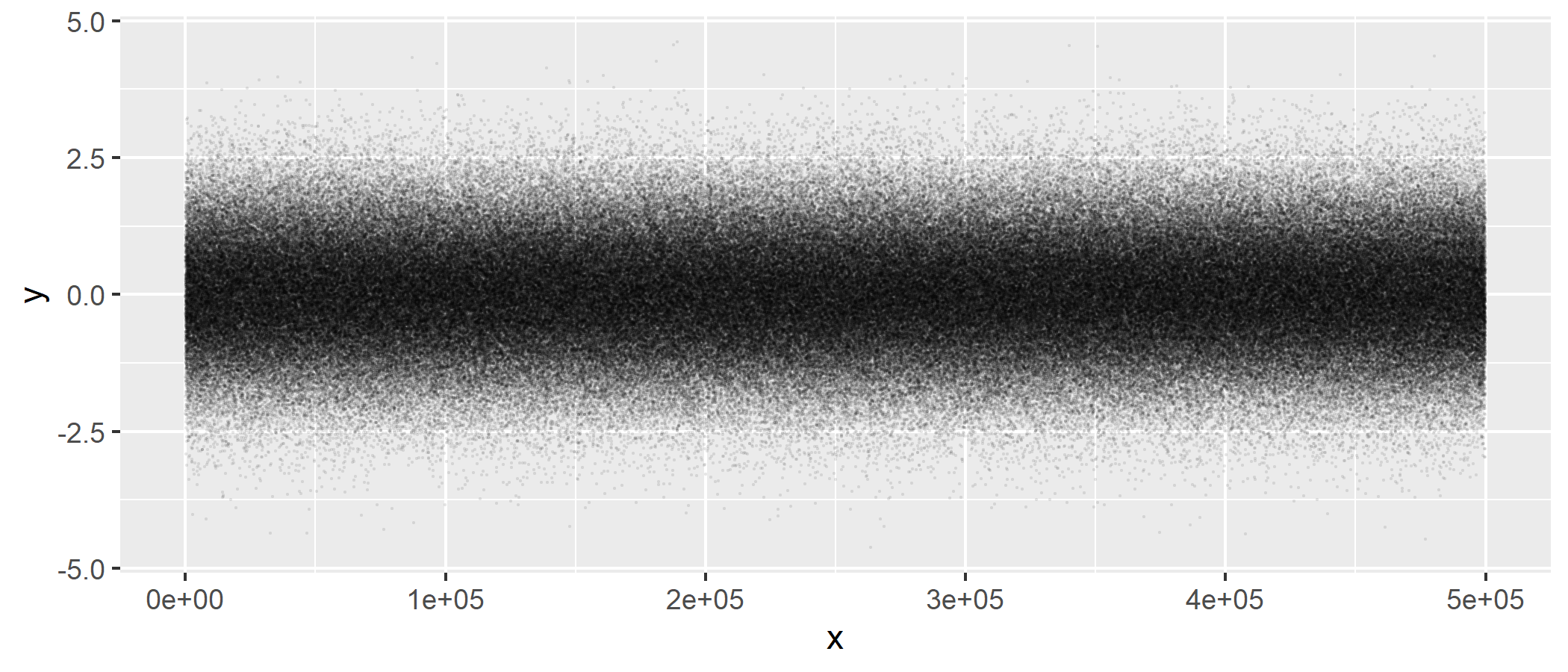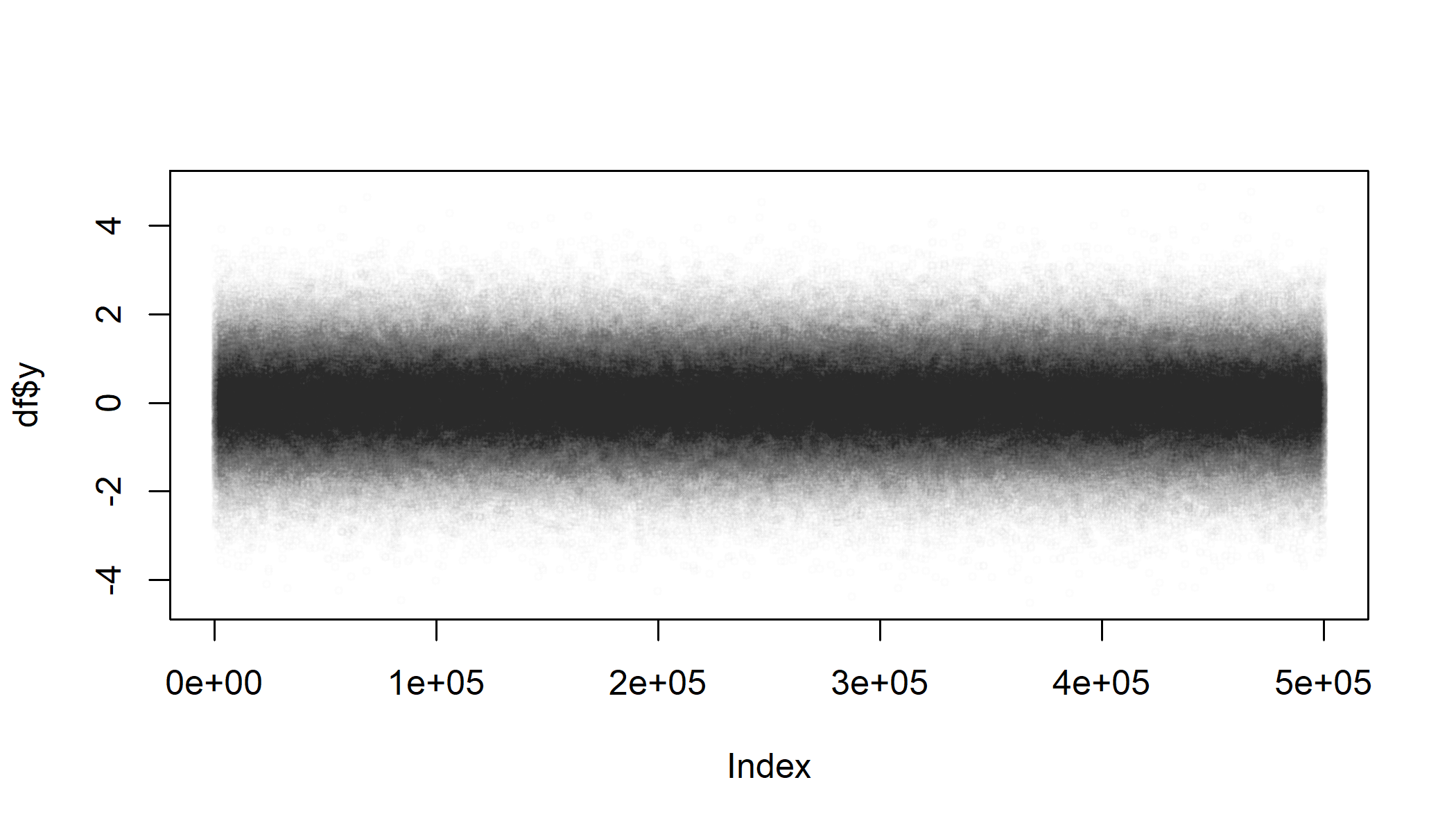Plotting a lot of data can be awfully slow. Let’s render plots in a job so that we kan keep working in the meantime. To demonstrate, here’s a simple dataset with 500k rows which takes 10+ seconds to render:

n = 5e5
df = data.frame(x = seq_len(n), y = rnorm(n))

## Render a ggplot2

ggplot2 is fast at building the plot “grammar” but rendering can be slow. So let’s build the plot in our main session but offload the rendering to a job::job(). We need to render it to a file because the job has no viewer.

# Build plot "grammar"
library(ggplot2)
gg = ggplot(df, aes(x = x, y = y)) +
geom_point(size = 0.1, alpha = 0.05)

# Render in a job
job::job({
ggsave("gg_points.png", plot = gg, width = 7, height = 3)
})## Render a base plot

We can do the same for base plots:

job::job({
png(filename = "base_points.png", width = 7, height = 4, units = "in", res = 300)
plot(df\$y, cex = 0.5, col = rgb(0, 0, 0, alpha = 0.01))
dev.off()
})